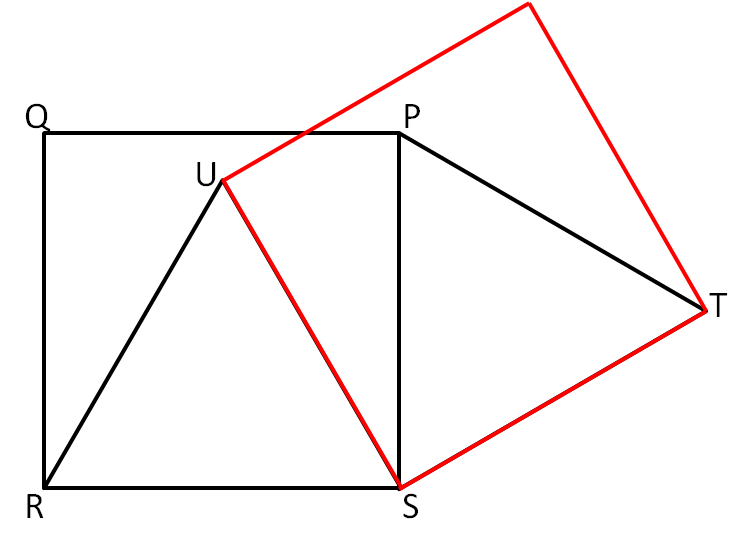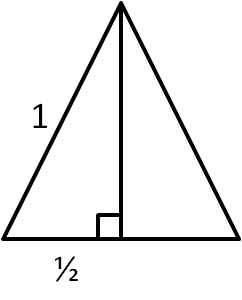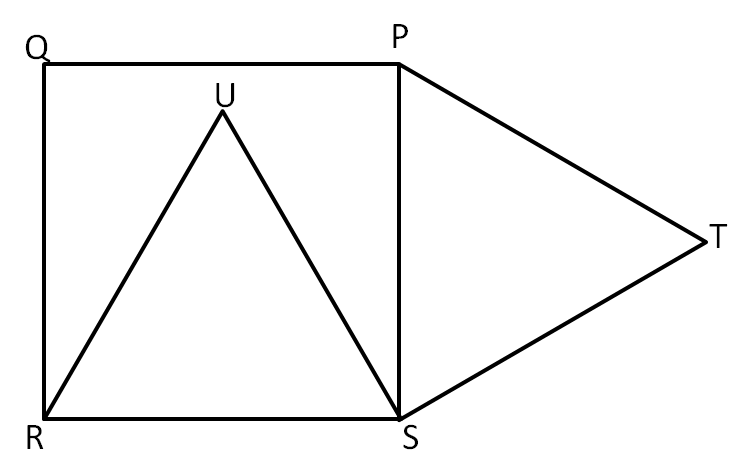#### You may also like### Some(?) of the Parts

A circle touches the lines OA, OB and AB where OA and OB are perpendicular. Show that the diameter of the circle is equal to the perimeter of the triangle### Triangle Midpoints

You are only given the three midpoints of the sides of a triangle. How can you construct the original triangle?### Fermat's Poser

Find the point whose sum of distances from the vertices (corners) of a given triangle is a minimum.

# Internal - External

##### Age 14 to 16 Short Challenge Level:

There are a number of different approaches that can be used to solve this problem.Rotation
Consider rotating the square $60^\circ$ clockwise about $S$.
Since $PQRS$ is a square, and $PST$ and $RSU$ are equilateral, $SR = SU = SP = ST = 1$.

As $\angle PST = 60^\circ$ (as the interior angle of an equilateral triangle), $P$ rotates onto $T$.
As $\angle RSU = 60^\circ$ (as the interior angle of an equilateral triangle), $R$ rotates onto $U$.

This means that the distance $UT$ is the same as $PR$, since distances are not changed by rotation.

Then, as $\angle PSR = 90^\circ$, Pythagoras' theorem can be used on triangle $PSR$ to find that:
$PR = \sqrt{1^2+1^2} = \sqrt{2}$.

Hence, $UT= PR = \sqrt{2}$.

Right Angle
$\angle PSR = 90^\circ$, as it is in a square.
$\angle USR = \angle TSP = 60^\circ$, as they are in equilateral triangles.

Then, $\angle PSU = 90^\circ - 60^\circ = 30^\circ$.
$\angle TSU = 60^\circ + 30^\circ = 90^\circ$.

Thus, TSU is a right angled triangle, so using Pythagoras' theorem: $UT = \sqrt{1^2+1^2} = \sqrt{2}$Coordinates
The height of an equilateral triangle of side length $1$ can be calculated to be, using Pythagoras' theorem:
$\sqrt{1^2 - \left(\frac{1}{2}\right)^2} = \frac{\sqrt{3}}{2}$

An equilateral triangle of side length $1$ has height $\frac{\sqrt{3}}{2}$.Let $R$ be the origin.
The coordinates of $U$ are $\left(\frac{1}{2},\frac{\sqrt{3}}{2} \right)$.
The coordinates of $T$ are $\left( 1 + \frac{\sqrt{3}}{2},\frac{1}{2} \right)$.

Then:
$UT = \sqrt{\left(1 + \frac{\sqrt{3}}{2} - \frac{1}{2}\right)^2 + \left( \frac{\sqrt{3}}{2} - \frac{1}{2} \right)^2}$
$= \sqrt{\left(\frac{3}{4} + \frac{2\sqrt{3}}{4} + \frac{1}{4}\right) + \left(\frac{3}{4} - \frac{2\sqrt{3}}{4} + \frac{1}{4}\right)}$
$=\sqrt{2}$
This problem is taken from the UKMT Mathematical Challenges.
You can find more short problems, arranged by curriculum topic, in our short problems collection.